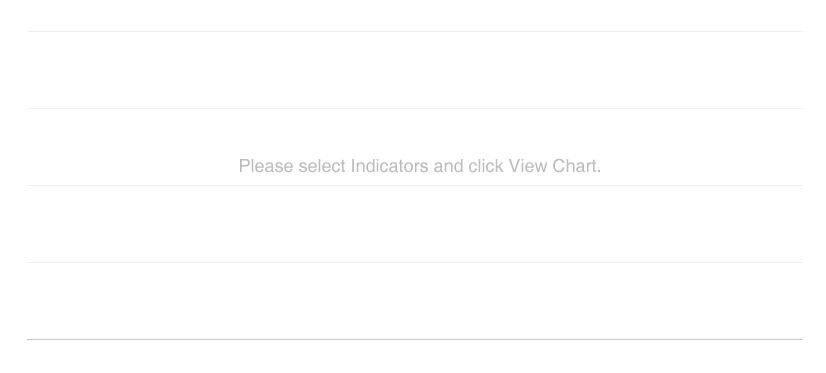# Chart Generator

Years Range :
Gross Income (Rs. Mn.)
Average Profit per Employee (Rs. Mn)
Average Assets per Employee (Rs. Mn)
Interest Bearing Liabilities (Rs. Mn.)
Interest Earning Assets (Rs. Mn.)
Total Deposits (Rs. Mn.)
Profit after taxation (Rs. Mn.)
Profit Before Taxation (Rs. Mn.)
Total Assets (Rs. Mn.)
Gross Dividend Paid (Rs. Mn.)
Gross NPA Ratio (%)
Return on Equity (%)
Return on Assets (%)
Cost to Income Ratio (%)
Dividend Per Share (Rs.)
Earnings Per Share (Rs.)
Market Value Per Share (Rs.)
Net Assets Value per Share (Rs.)
Shareholders` Funds (Rs.Bn.)
Market capitalisation (Rs. Bn.)
No. of Employees
No. of ATMsTOP
Close